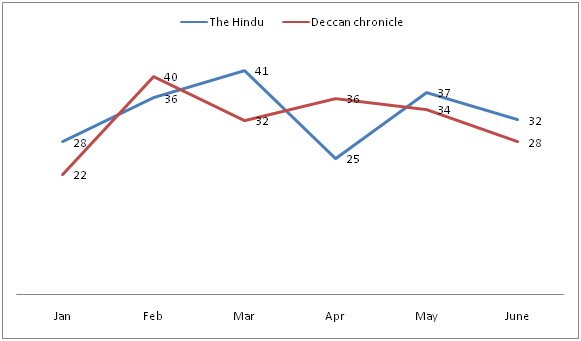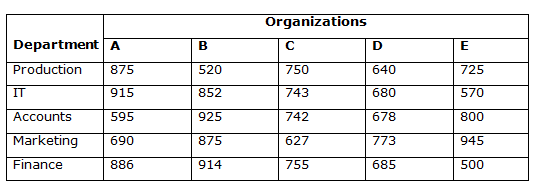# Quantitative Aptitude Questions (Data Interpretation) for RRB Assistant Mains 2018 Day-209

Dear Readers, RRB Assistant is conducting Online Examination for the recruitment of Assistant. To enrich your preparation here we have providing new series of Data Interpretation – Quantitative Aptitude Questions. Candidates those who are appearing in RRB Assistant Mains Exams can practice these Quantitative Aptitude average questions daily and make your preparation effective.

[WpProQuiz 3881]

Directions (Q. 1 – 5) Study the following information carefully and answer the given questions:

The following line graph shows the total number of readers (In thousands) of two different newspapers in different months in the year 2016.1) If the total number of Deccan chronicle readers in July 2016 is equal to the average number of Deccan chronicle readers in April and May 2016 together and the total number of The Hindu readers in July 2016 is 1/5 of total number of Deccan chronicle readers in July 2016, then find the total number of The Hindu readers in July 2016?

a) 7000

b) 8500

c) 6500

d) 9000

e) None of these

2) If the total number of The Hindu and Deccan chronicle readers in April 2017 is increased by 20 % and 25 % respectively while comparing with the previous year April month, then find the sum of total number of The Hindu readers and Deccan chronicle readers in April 2017?

a) 84000

b) 75000

c) 68000

d) 92000

e) None of these

3) Find the difference between the total number of The Hindu readers in the month of Feb, Mar, May and June together to that of Deccan chronicle readers in the month of Jan, Mar, Apr and June together?

a) 32000

b) 41000

c) 28000

d) 24000

e) None of these

4) Total number of The Hindu readers of all the given months together is approximately what percentage of Deccan chronicle readers of all the given months together?

a) 98 %

b) 86 %

c) 112 %

d) 104 %

e) 121 %

5) Find the ratio between the total number of The Hindu readers in the month of Jan, mar and May together to that of total number of Deccan chronicle readers in the month of Feb, Apr and June together?

a) 51: 55

b) 53 : 52

c) 38 : 27

d) 26 : 23

e) None of these

Directions (Q. 6 – 10) Study the following information carefully and answer the given questions:

The following table shows the total number of people working in various departments of different organizations in particular city.6) Total number of employees working in Accounts department is approximately what percentage of total number of employees working in marketing department of all the given organizations?

a) 84 %

b) 96 %

c) 75 %

d) 108 %

e) 117 %

7) Find the ratio between the total number of employees working in organization C to that of D of all the given department together?

a) 1156: 1027

b) 2458: 2175

c) 987: 756

d) 3617: 3456

e) None of these

8) Which of the following Organization has highest number of employees?

a) Organization A

b) Organization B

c) Organization C

d) Organization D

e) Organization E

9) Find the difference between the total number of employees working in Organization B to that of E of all the given department together?

a) 546

b) 678

c) 424

d) 712

e) None of these

10) Total number of employees working in IT and Finance of Organization A, C and E together is approximately what percentage more/less than the total number of employees working in Production and Marketing of Organization B, D and E together?

a) 7 % more

b) 9 % less

c) 2 % less

d) 14 % more

e) 16 % less

Direction (1-5) :

Explanation:

The total number of Deccan chronicle readers in July 2016

= > (36000 + 34000)/2

= > 35000

The total number of The Hindu readers in July 2016

= > (1/5)*35000

= > 7000

The total number of The Hindu readers in July 2016 = 7000

Explanation:

The total number of The Hindu readers in April 2017

= > 25*(120/100) = 30 thousand

The total number of Deccan chronicle readers in April 2017

= > 36*(125/100) = 45 thousand

Required sum = 30000 + 45000 = 75000

Explanation:

The total number of The Hindu readers in the month of Feb, Mar, May and June

= > (36 + 41 + 37 + 32) thousand

= > 146000

The total number of Deccan chronicle readers in the month of Jan, Mar, Apr and June

= > (22 + 32 + 36 + 28) thousand

= > 118000

Required difference = 146000 – 118000 = 28000

Explanation:

Total number of The Hindu readers of all the given months together

= > (28 + 36 + 41 + 25 + 37 + 32) thousand).

= > 199000

Total number of Deccan chronicle readers of all the given months together

= > (22 + 40 + 32+ 36 + 34+ 28) thousand

= > 192000

Required % = (199000/192000)*100 = 103.64 = 104 %

Explanation:

The total number of The Hindu readers in the month of Jan, mar and May together

= > (28 + 41 + 37) thousand

= > 106000

The total number of Deccan chronicle readers in the month of Feb, Apr and June together

= > (40 + 36 + 28) thousand

= > 104000

Required ratio = 106000 : 104000 = 53 : 52

Direction (6-10) :

Explanation:

Total number of employees working in Accounts department

= > 595 + 925 + 742 + 678 + 800

= > 3740

Total number of employees working in marketing department

= > 690 + 875 + 627 + 773 + 945

= > 3910

Required %= (3740/3910)*100 = 95.65 % = 96 %

Explanation:

The total number of employees working in organization C

= > 750 + 743 + 742 +627 + 755

= > 3617

The total number of employees working in organization D

= > 640 + 680 + 678 + 773 + 685

= > 3456

Required ratio = 3617: 3456

Explanation:

Total number of employees in Organization A

= > 875 + 915 + 595 + 690 + 886

= > 3961

Total number of employees in Organization B

= > 520 + 852 + 925 + 875 + 914

= > 4086

Total number of employees in Organization C

= > 750 + 743 + 742 +627 + 755

= > 3617

Total number of employees in Organization D

= > 640 + 680 + 678 + 773 + 685

= > 3456

Total number of employees in Organization E

= > 725 + 570 + 800 + 945 + 500

= > 3540

Organization B has highest number of employees.

Explanation:

The total number of employees working in Organization B

= > 520 + 852 + 925 + 875 + 914

= > 4086

The total number of employees working in Organization E

= > 725 + 570 + 800 + 945 + 500

= > 3540

Required difference = 4086 – 3540 =546

Explanation:

Total number of employees working in IT and Finance of Organization A, C and E together

= > 915 + 743 + 570 + 886 + 755 + 500

= > 4369

Total number of employees working in Production and Marketing of Organization B, D and E together

= > 520 + 640 + 725 + 875 +773 + 945

= > 4478

Required % = [(4478 – 4369)/4478]*100 = (109/4478)*100

= > 2.434 % = 2 % less

Daily Practice Test Schedule | Good Luck

 Topic Daily Publishing Time Daily News Papers & Editorials 8.00 AM Current Affairs Quiz 9.00 AM Quantitative Aptitude “20-20” 11.00 AM Vocabulary (Based on The Hindu) 12.00 PM General Awareness “20-20” 1.00 PM English Language “20-20” 2.00 PM Reasoning Puzzles & Seating 4.00 PM Daily Current Affairs Updates 5.00 PM Data Interpretation / Application Sums (Topic Wise) 6.00 PM Reasoning Ability “20-20” 7.00 PM English Language (New Pattern Questions) 8.00 PM